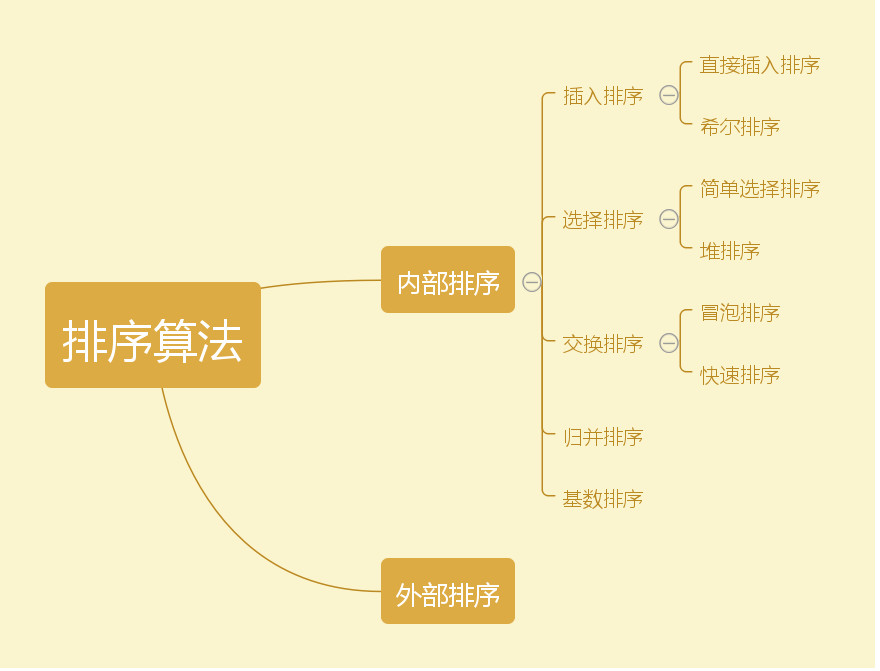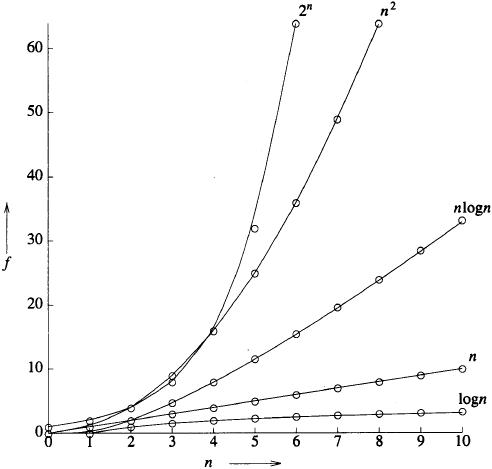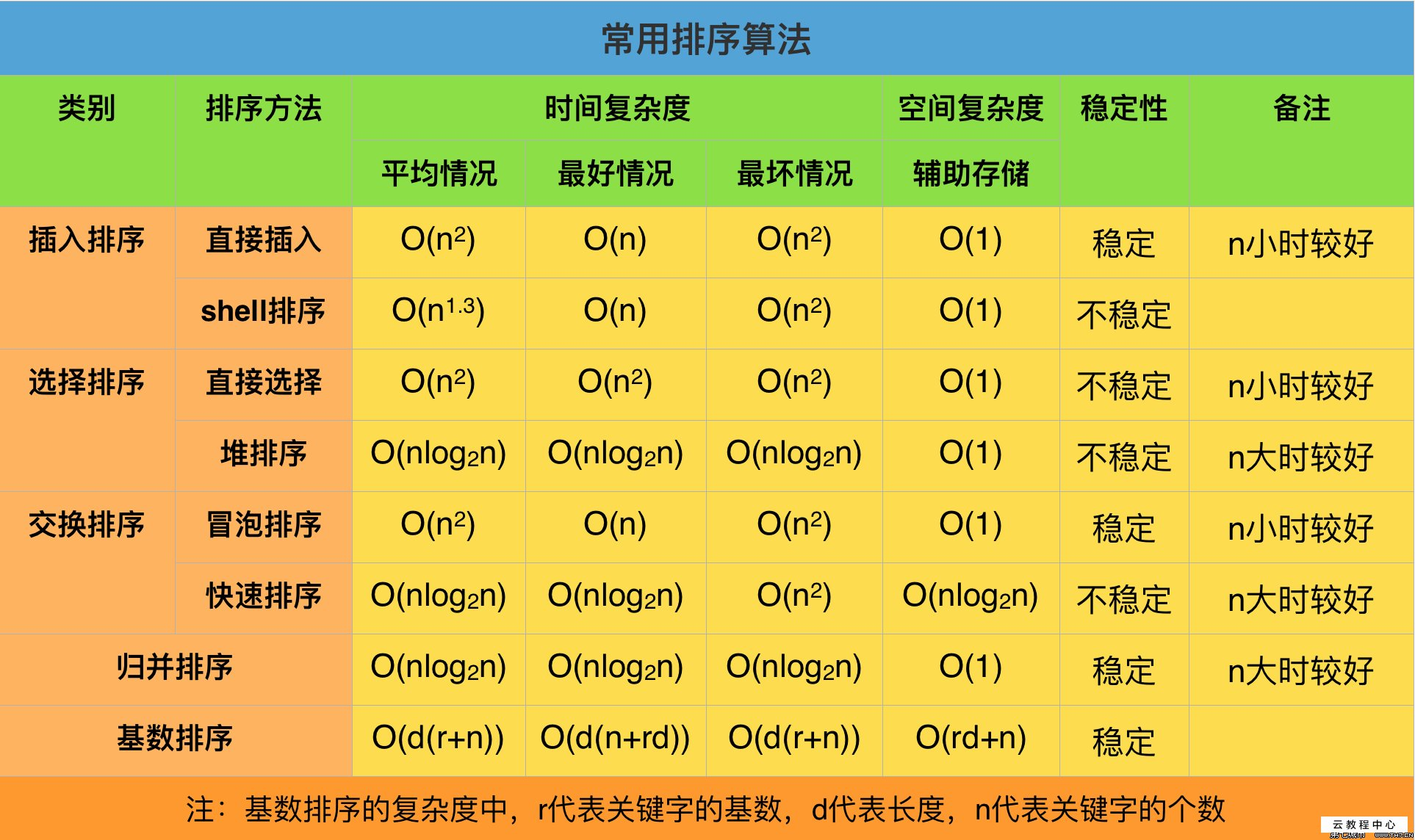# 算法入门基础

2019/04/10 10:10

## 排序分类：

• ### 常见分类如图：## 时间复杂度：

• ### 概述：

时间复杂度是一个函数，它定性描述该算法的运行时间。这是一个代表算法输入值的字符串的长度的函数。时间复杂度常用大O符号表述，不包括这个函数的低阶项和首项系数。使用这种方式时，时间复杂度可被称为是渐近的，亦即考察输入值大小趋近无穷时的情况。

• ### 时间频度

一个语句执行次数称为语句频度或时间频度，记为T(n)。算法花费的时间与算法中语句的执行次数成正比例，哪个算法中语句执行次数多，它花费时间就多。

• ### 时间复杂度：

一般情况下，算法中基本操作重复执行的次数是问题规模n的某个函数，用T(n)表示，若有某个辅助函数f(n),使得当n趋近于无穷大时，T（n)/f (n)的极限值为不等于零的常数，则称f(n)是T(n)的同数量级函数。记作T(n)=O(f(n)),称O(f(n)) 为算法的渐进时间复杂度，简称时间复杂度

举个栗子：

int sum = 0;
int b = 100;
for(int i = 0; i<= b;i++){
sum += i;
}
//  T(n) = n+1 = 100+1

sum = (1+b)*b/2 //同样也可以计算出结果，但是T(n) = 1


简而言之，在T(n)=O(f(n))中，当n趋近无穷大时，有 T(n)/f(n) = c ,极限值c是一个不为0 的常数，就叫 f(n) 是T(n)的同量级函数，若求得 f(n) = n ,则O(f(n)) =O(n) .

• ### 各时间复杂度增长曲线：• ### 各算法时间复杂度：0
0 收藏

### 作者的其它热门文章0 评论
0 收藏
0